Computational Methods

Four methods of estimation can be specified in the PROC VARCOMP statement by using the METHOD= option. They are described in the following sections.

### The Type I Method

This method (METHOD=TYPE1) computes the Type I sum of squares for each effect, equates each mean square involving only random effects to its expected value, and solves the resulting system of equations (Gaylor, Lucas, and Anderson; 1970). The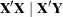matrix is computed and adjusted in segments whenever memory is not sufficient to hold the entire matrix.

### The MIVQUE0 Method

Based on the technique suggested by Hartley, Rao, and LaMotte (1978), the MIVQUE0 method (METHOD=MIVQUE0) produces unbiased estimates that are invariant with respect to the fixed effects of the model and that are locally best quadratic unbiased estimates given that the true ratio of each component to the residual error component is zero. The technique is similar to TYPE1 except that the random effects are adjusted only for the fixed effects. This affords a considerable timing advantage over the TYPE1 method; thus, MIVQUE0 is the default method used in PROC VARCOMP. The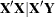matrix is computed and adjusted in segments whenever memory is not sufficient to hold the entire matrix. Each element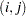of the form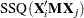is computed, where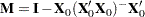and where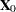is part of the design matrix for the fixed effects,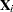is part of the design matrix for one of the random effects, and SSQ is an operator that takes the sum of squares of the elements. For more information refer to Rao (1971, 1972) and Goodnight (1978).

### The Maximum Likelihood Method

The maximum likelihood method ( METHOD=ML) computes maximum likelihood estimates of the variance components; refer to Searle, Casella, and McCulloch (1992). The computing algorithm makes use of the W-transformation developed by Hemmerle and Hartley (1973) and Goodnight and Hemmerle (1979). The procedure uses a Newton-Raphson algorithm, iterating until the log-likelihood objective function converges.

The objective function for METHOD=ML is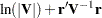, where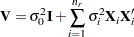and where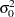is the residual variance,is the number of random effects in the model,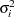represents the variance components,is part of the design matrix for one of the random effects, and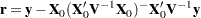is the vector of residuals.

### The Restricted Maximum Likelihood Method

The restricted maximum likelihood method ( METHOD=REML) is similar to the maximum likelihood method, but it first separates the likelihood into two parts: one that contains the fixed effects and one that does not (Patterson and Thompson; 1971). The procedure uses a Newton-Raphson algorithm, iterating until convergence is reached for the log-likelihood objective function of the portion of the likelihood that does not contain the fixed effects. Using notation from earlier methods, the objective function for METHOD=REML is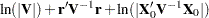. Refer to Searle, Casella, and McCulloch (1992) for additional details.

### The GRR Method

Based on the technique suggested by Burdick, Borror, and Montgomery (2005), the GRR method (METHOD=GRR) produces minimum variance unbiased estimators.